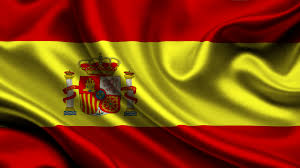# Spain Mathematical Olympiad Problem 3Find the largest integer $N$ satisfying the following two conditions:

(i) $\lfloor \frac N3 \rfloor$ consists of three equal digits;

(ii) $\lfloor \frac N3 \rfloor = 1 + 2 + 3 +\cdots + n$ for some positive integer $n.$

$\lfloor \cdot \rfloor$ denotes the floor function.

×

Problem Loading...

Note Loading...

Set Loading...